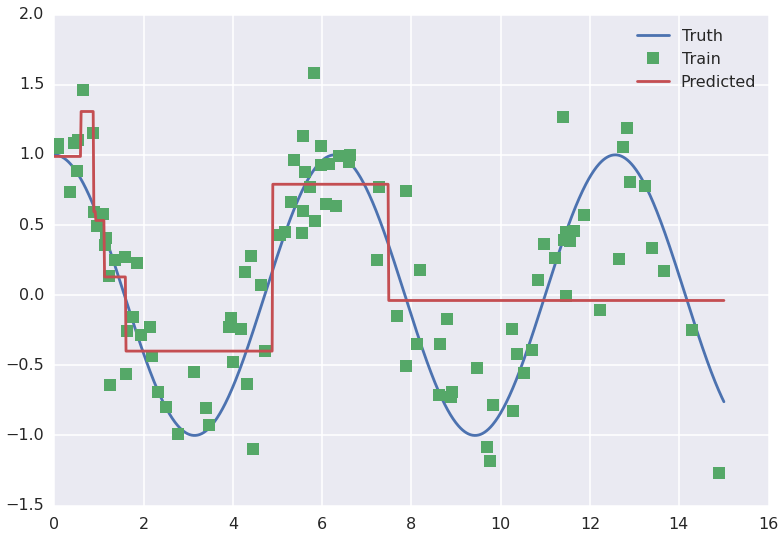In :
!date
import numpy as np, pandas as pd, matplotlib.pyplot as plt, seaborn as sns
%matplotlib inline
sns.set_context('poster')
sns.set_style('darkgrid')

Fri Feb 13 15:29:21 PST 2015

In :
# set random seed for reproducibility
np.random.seed(12345)

In :
# simulate some data from a familiar distribution
x_true = np.linspace(0,15,1000)
y_true = np.cos(x_true)

sigma_true = .3
x_train = np.random.choice(x_true, size=100)
y_train = np.random.laplace(np.cos(x_train), sigma_true)

In :
# load the decision tree module of sklearn
import sklearn.tree

# make a DecisionTreeRegressor
dt = sklearn.tree.DecisionTreeRegressor(max_depth=3)

# fit it to the simulated training data
X_train = x_train[:,None]
dt.fit(X_train, y_train)

# predict for a range of x values
X_true = x_true[:,None]  # horrible, but remember it!
y_pred = dt.predict(X_true)

In :
# have a look
plt.plot(x_true, y_true, '-', label='Truth')
plt.plot(x_train, y_train, 's', label='Train')
plt.plot(x_true, y_pred, '-', label='Predicted')
plt.legend()

Out:
<matplotlib.legend.Legend at 0x2b2c8595ab90>In :
# tricky, since it uses recursion

def print_tree(t, root=0, depth=1):
if depth == 1:
print 'def predict(X_i):'
indent = '    '*depth
print indent + '# node %s: impurity = %.2f' % (str(root), t.impurity[root])
left_child = t.children_left[root]
right_child = t.children_right[root]

if left_child == sklearn.tree._tree.TREE_LEAF:
print indent + 'return %s # (node %d)' % (str(t.value[root]), root)
else:
print indent + 'if X_i[%d] < %.2f: # (node %d)' % (t.feature[root], t.threshold[root], root)
print_tree(t, root=left_child, depth=depth+1)

print indent + 'else:'
print_tree(t,root=right_child, depth=depth+1)

print_tree(dt.tree_)

def predict(X_i):
# node 0: impurity = 0.46
if X_i < 1.11: # (node 0)
# node 1: impurity = 0.08
if X_i < 0.88: # (node 1)
# node 2: impurity = 0.04
if X_i < 0.59: # (node 2)
# node 3: impurity = 0.02
return [[ 0.98872712]] # (node 3)
else:
# node 4: impurity = 0.02
return [[ 1.30906617]] # (node 4)
else:
# node 5: impurity = 0.00
if X_i < 0.92: # (node 5)
# node 6: impurity = 0.00
return [[ 0.59581346]] # (node 6)
else:
# node 7: impurity = 0.00
return [[ 0.53263382]] # (node 7)
else:
# node 8: impurity = 0.43
if X_i < 4.89: # (node 8)
# node 9: impurity = 0.18
if X_i < 1.59: # (node 9)
# node 10: impurity = 0.13
return [[ 0.12991532]] # (node 10)
else:
# node 11: impurity = 0.14
return [[-0.39874553]] # (node 11)
else:
# node 12: impurity = 0.45
if X_i < 7.48: # (node 12)
# node 13: impurity = 0.09
return [[ 0.79041947]] # (node 13)
else:
# node 14: impurity = 0.41
return [[-0.03795854]] # (node 14)


Copy-and-paste, you have a pure python version of the decision tree classifier:

In :
def predict(X_i):
# node 0: impurity = 0.46
if X_i < 1.11: # (node 0)
# node 1: impurity = 0.08
if X_i < 0.88: # (node 1)
# node 2: impurity = 0.04
if X_i < 0.59: # (node 2)
# node 3: impurity = 0.02
return [[ 0.98872712]] # (node 3)
else:
# node 4: impurity = 0.02
return [[ 1.30906617]] # (node 4)
else:
# node 5: impurity = 0.00
if X_i < 0.92: # (node 5)
# node 6: impurity = 0.00
return [[ 0.59581346]] # (node 6)
else:
# node 7: impurity = 0.00
return [[ 0.53263382]] # (node 7)
else:
# node 8: impurity = 0.43
if X_i < 4.89: # (node 8)
# node 9: impurity = 0.18
if X_i < 1.59: # (node 9)
# node 10: impurity = 0.13
return [[ 0.12991532]] # (node 10)
else:
# node 11: impurity = 0.14
return [[-0.39874553]] # (node 11)
else:
# node 12: impurity = 0.45
if X_i < 7.48: # (node 12)
# node 13: impurity = 0.09
return [[ 0.79041947]] # (node 13)
else:
# node 14: impurity = 0.41
return [[-0.03795854]] # (node 14)

In :
predict(X_true)

Out:
[[0.98872712]]
In [ ]: